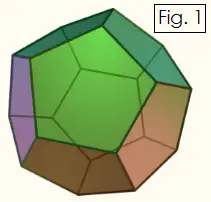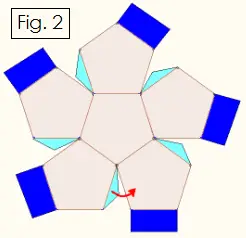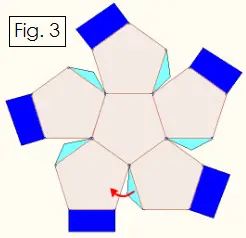# Constructing Geometric Solids:Dodecahedron

A dodecahedron (or cubocta) is a 12-sided solid figure in which each face is a regular pentagon. Each vertex is the intersection of three pentagons. Along with the cube, tetrahedron, octahedron, and icosahedron, the dodecahedron is one of the five Platonic solids. The dodecahedron is called the "dual" of the icosahedron because each face of the dodecahedron corresponds to the vertices of the icosahedron and vice versa.

You can construct a dodecahedron from cardstock and glue using the instructions and pattern described below.Look at the Figure 1 on the right to see how a dodecahedron is constructed from regular pentagons. We will construct the dodecahedron by making two halves with six pentagons, then glue the two halves together.Cut out the pattern shown in Figure 2. Fold all the pentagons and dark blue tabs upward so that the figure curls inward toward the center. Fold the light blue tabs upward, and glue each one to the adjacent pentagon. When the glue dries, you will have a bowl shape with the tabs glued on the inside. Do not glue the dark blue tabs yet.

Next, cut out the shape shown below in Figure 3, the mirror image of Figure 2. Repeat the same procedure as above.Put a dab of glue on each of the 10 dark blue tabs, and fit the two halves of the solid together. The dark blue tabs will interlock with the edges of the pentagons, and the tabs will be tucked inside the finished dodecahedron.